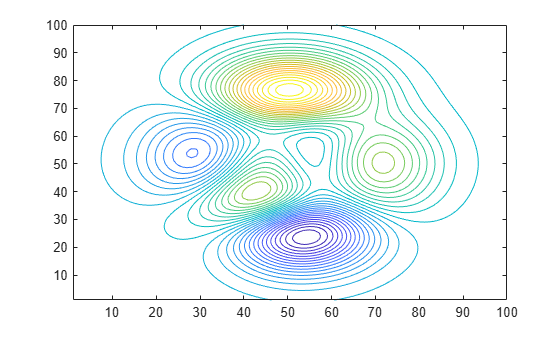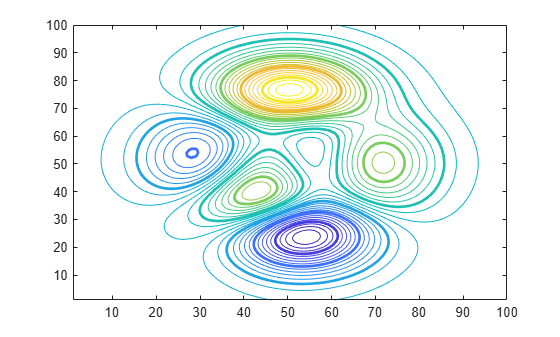Documentation

Highlight Specific Contour Levels

This example shows how to highlight contours at particular levels.

Define Z as the matrix returned from the peaks function.

Z = peaks(100);

Round the minimum and maximum data values in Z and store these values in zmin and zmax, respectively. Define zlevs as 40 values between zmin and zmax.

zmin = floor(min(Z(:)));
zmax = ceil(max(Z(:)));
zinc = (zmax - zmin) / 40;
zlevs = zmin:zinc:zmax;

Plot the contour lines.

figure
contour(Z,zlevs)Define zindex as a vector of integer values between zmin and zmax indexed by 2.

zindex = zmin:2:zmax;

Retain the previous contour plot. Create a second contour plot and use zindex to highlight contour lines at every other integer value. Set the line width to 2.

hold on
contour(Z,zindex,'LineWidth',2)
hold off